Test cases

The data for the estimation of the discretization error/uncertainty of functional and local flow quantities was obtained from six statistically steady, two-dimensional flows of an incompressible fluid computed with the open-usage viscous flow code ReFRESCO (www.refresco.org):

·       Flow over a flat plate at three different Reynolds numbers based on the plate length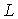, incoming velocity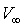and fluid kinematic viscosity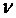,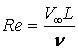.

I.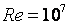.

II.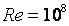.

III.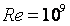.

·       Flow around a NACA 0012 airfoil at Reynolds number based on the airfoil chord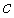, incoming velocityand fluid kinematic viscosityof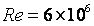at three angles of attack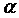:

IV.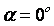.

V.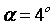.

VI.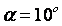.

For these six cases, simulations were performed with three isotropic eddy-viscosity turbulence models:

a.    The one-equation model of Spalart & Allmaras in the formulation without the tripping term (SAnoft2).

b.    The Shear-Stress Transport (SST) k-w two-equation model.

c.    The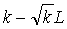two-equation model.

Different cases available will be designated according to the numbering presented above. For example, CASE IVb corresponds to the flow around the NACA 0012 airfoil atsimulated with the SST k-w two-equation model.

All calculations were performed using double precision (15 digits) and iterative convergence criteria based on the maximum (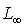norm) normalized residual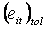of all transport equations solved for each flow condition. Normalized residuals are equivalent to the change of dimensionless dependent variables in a simple Jacobi iteration. Reference values are the fluid density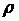,andfor the plate flow and,andfor the flow around the airfoils.  Maximum values offor the six test cases are given below.

 Test Case Flat Plate NACA 0012 I II III IV V VI10-8 10-8 5×10-8 10-8 2×10-8 2×10-8